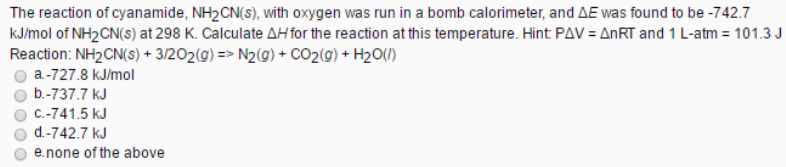# Problem: The reaction of cyanamide, NH2CN(s), with oxygen was run in a bomb calorimeter, and ΔE was found to be -742.7 kJ/mol of NH2CN(s) at 298 K. Calculate ΔH for the reaction at this temperature. Reaction: NH2CN (s) + 3/2O2 (g) → N2 (g) + CO2 (g) + H2O (l) a. -727.8 kJ/mol b. -737.7 kJ c. -741.5 kJ d. -742.7 kJ e. none of the above

###### FREE Expert Solution
94% (351 ratings)###### Problem Details

The reaction of cyanamide, NH2CN(s), with oxygen was run in a bomb calorimeter, and ΔE was found to be -742.7 kJ/mol of NH2CN(s) at 298 K. Calculate ΔH for the reaction at this temperature.

Reaction: NH2CN (s) + 3/2O2 (g) → N2 (g) + CO2 (g) + H2O (l)

a. -727.8 kJ/mol

b. -737.7 kJ

c. -741.5 kJ

d. -742.7 kJ

e. none of the aboveFrequently Asked Questions

What scientific concept do you need to know in order to solve this problem?

Our tutors have indicated that to solve this problem you will need to apply the Thermochemical Equations concept. You can view video lessons to learn Thermochemical Equations. Or if you need more Thermochemical Equations practice, you can also practice Thermochemical Equations practice problems.